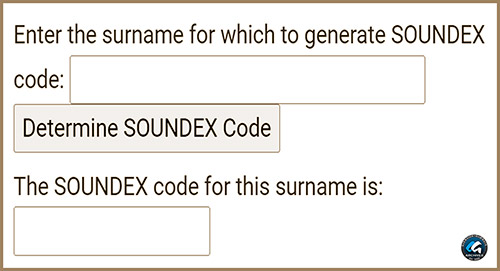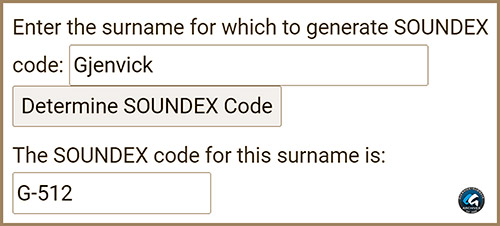# SOUNDEX Code GeneratorSoudex Code Generator Coded with JavaScript to Produce the Soundex Code for any English Name (i.e. No Special Characters). GGA Image ID # 1cf95a18cdSample Soundex Code Results for the Surname "Gjenvick," which is "G-512." GGA Image ID # 1cf9749e6c

SOUNDEX is a phonetic code utilized to index various U.S. Census data since 1880.

Instructions:

1. Enter the surname for which to generate SOUNDEX code.
2. Click on the SOUNDEX button.
3. See the corresponding SOUNDEX code in the field.

Enter the surname for which to generate SOUNDEX code:

The SOUNDEX code for this surname is:

## Soundex Code

function initMail(form) {
form.message.value = "Hi " + form.sendto.value + " (" + form.to.value + "):\n\n"
+ text + "\n\nYour Friend,\n" + form.sendername.value + "(" +
form.senderemail.value + ")";
return (form.to.value != "");
}
// End -->
<script language=JavaScript>
<!-- hide this code from non-scriptable browsers

// create object listing the SOUNDEX values for each letter
// -1 indicates that the letter is not coded, but is used for coding
// 1 is for BFPV
// 2 is for CGJKQSXZ
// 3 is for DT
// 4 is for L
// 5 is for M
// 6 is for R
function makesoundex() {
this.a = -1
this.b = 1
this.c = 2
this.d = 3
this.e = -1
this.f = 1
this.g = 2
this.h = -1
this.i = -1
this.j = 2
this.k = 2
this.l = 4
this.m = 5
this.n = 5
this.o = -1
this.p = 1
this.q = 2
this.r = 6
this.s = 2
this.t = 3
this.u = -1
this.v = 1
this.w = -1
this.x = 2
this.y = -1
this.z = 2
}

var sndx=new makesoundex()

// check to see that the input is valid
function isSurname(name) {
if (name=="" || name==null) {
return false
} else {
for (var i=0; i<name.length; i++) {
var letter=name.charAt(i)
if (!(letter>='a' && letter<='z' || letter>='A' && letter<='Z')) {
return false
}
}
}
return true
}

// Collapse out directly adjacent sounds
// 1. Assume that surname.length>=1
// 2. Assume that surname contains only lowercase letters
function collapse(surname) {
if (surname.length==1) {
return surname
}
var right=collapse(surname.substring(1,surname.length))
if (sndx[surname.charAt(0)]==sndx[right.charAt(0)]) {
return surname.charAt(0)+right.substring(1,right.length)
}
return surname.charAt(0)+right
}

// Compute the SOUNDEX code for the surname
function soundex(form) {
form.result.value=""
if (!isSurname(form.surname.value)) {
return
}
var stage1=collapse(form.surname.value.toLowerCase())
form.result.value+=stage1.charAt(0).toUpperCase() // Retain first letter
form.result.value+="-" // Separate letter with a dash
var stage2=stage1.substring(1,stage1.length)
var count=0
for (var i=0; i<stage2.length && count<3; i++) {
if (sndx[stage2.charAt(i)]>0) {
form.result.value+=sndx[stage2.charAt(i)]
count++
}
}
for (; count<3; count++) {
form.result.value+="0"
}

form.surname.select()
form.surname.focus()
}
// end code hiding -->
</script>
<script language="JavaScript">
function blockError(){return true;}
window.onerror = blockError;
</script>

Biographies

Entertainment

History

Genealogy

Sheet Music

Related Topics